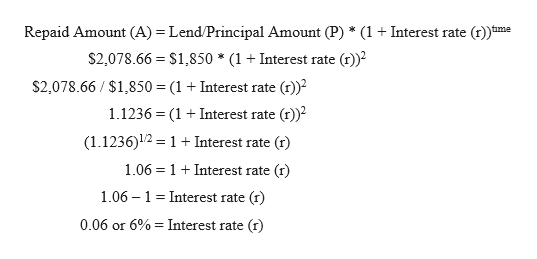Finding the Interest Rate: Concept Connection Example 6-3 (page 237) 18. What interest rates are implied by the following lending arrangements?a. You borrow \$500 and repay \$555 in one year.b. You lend \$1,850 and are repaid \$2,078.66 in two years.c. You lend \$750 and are repaid \$1,114.46 in five years with quarterly compounding.d. You borrow \$12,500 and repay \$21,364.24 in three years under monthly compounding. (Note. In parts c and d, be sure to give your answer as the annual nominal rate.)Lasher, William R.. Practical Financial Management (Page 276). South-Western College Pub. Kindle Edition.

Question

Finding the Interest Rate: Concept Connection Example 6-3 (page 237) 18. What interest rates are implied by the following lending arrangements?

a. You borrow \$500 and repay \$555 in one year.

b. You lend \$1,850 and are repaid \$2,078.66 in two years.

c. You lend \$750 and are repaid \$1,114.46 in five years with quarterly compounding.

d. You borrow \$12,500 and repay \$21,364.24 in three years under monthly compounding. (Note. In parts c and d, be sure to give your answer as the annual nominal rate.)

Lasher, William R.. Practical Financial Management (Page 276). South-Western College Pub. Kindle Edition.

Step 1

Interest rate:

An interest rate is a percentage on the principal amount at which a lender gives money to a borrower and it’s an excess amount on the principal amount. It is generally represented as an annual rate.

Compounding Interest:

It is the method for computing interest at the initial amount of principal which includes the previous period’s accumulated interest. It can be compounded annually, half-yearly, quarterly and daily. The rate of compounding interest depends on the frequency of compounding.

Formula for the calculation of Amount (A) using Compounded Interest:

Amount (A) = Principal Amount (P) * (1 + rate of interest (r) / Number of times compounded (n))n*time period

Step 2

(a)

Given,

Loan/Principal Amount (P) = \$500

Repay Amount (A) = \$555

Time in years (t) = 1

Type of compounding interest = Annually

Calculation of Interest rate:help_outlineImage TranscriptioncloseRepay Amount (A) Loan/Principal Amount (P) * (1 Interest rate () fime () \$555 \$500 * (1 +Interest rate \$555 \$5001 + Interest rate (r) 1.11 1Interest rate (r) 1.11 1 Interest rate (r) - 0.11 or 11% = Interest rate (t) fullscreen
Step 3

(b)

Given,

Lend/Principal Amount (P) = \$1,850

Repaid Amount (A) = \$2,078.66

Time in years (t) = 2

Type of c...help_outlineImage Transcriptionclose(1)t Repaid Amount (A) Lend/Principal Amount (P) * (1 + Interest rate fime \$2,078.66 \$1,850 * (1 + Interest rate ()) \$2,078.66 \$1,850 (1Interest rate (t) () (1Interest rate 1.1236 (1.1236)12 1 +Interest rate () 1.06 1Interest rate (r) 1.06 1 Interest rate (r) 0.06 or 6% Interest rate (r) fullscreen

Want to see the full answer?

See Solution

Want to see this answer and more?

Our solutions are written by experts, many with advanced degrees, and available 24/7

See Solution
Tagged in